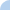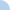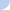Q3P20 Multiply & Divide Mixed Numbers

Make sure that you copy these problems onto your Solutions Sheet.

Then, change any mixed number into an equivalent fraction and show how you would solve the problem.

 This quiz is timed. The total time allowed for this quiz is 6 minutes.Quiz Log In